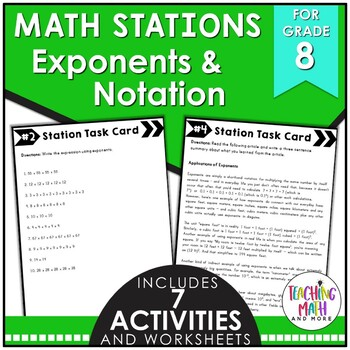## Wednesday, August 31, 2016

### Teaching Scientific Notation {Grade 8}

One of my favorite topics to teach in eighth grade math {Pre-Algebra} was Exponents & Scientific Notation. Students have prior knowledge of numbers and how they can be big or small, but have little grasp of how BIG and small they can really get. Giving students options with hands-on activities and Math stations lets them explore the concepts and gather their own understandings of the Math.

I have listed here four activities to review and practice Exponents & Scientific Notation. I hope these help jump start your lesson planning into Exponents & Scientific Notation. The key to getting your students involved in the learning process is to include interactive, visual, engaging activities for them to actively participate in.

These interactive notebook activities are intended to help students understand and apply the properties of integer exponents, use numbers expressed in single digit form times an integer power of 10, and to perform operations with scientific notation and use appropriate measurements.

Included are:
-4 different Exponents & Scientific Notation activities to engage students
-Teacher facilitated activity for 60-90 minutes of classroom time
-Activities include: Vocabulary, Writing expressions using exponents, Writing numbers in scientific notation, and Simplifying expressions with exponents.
-Examples of Completed Interactive Math Notebook Activities are includedThis math station activity is intended to help students understand and apply the properties of integer exponents, use numbers expressed in single digit form times an integer power of 10, and to perform operations with scientific notation and use appropriate measurements.

Included are:
-6 different stations to engage students
-Teacher facilitated activity for 60-90 minutes of classroom time
-Stations include:
1. Vocabulary
3. Practice of Exponents & Scientific Notation
4. Additional Practice of Exponents & Scientific Notation
5. Real World applications of Exponents & Scientific Notation
6. Word problems of Exponents & Scientific Notation
-Student Station Guide helps students record their answersThis lesson unit is intended to help you assess how well students are able to apply properties of integer exponents, express numbers in the form of a single digit times an integer power, and express how many times as much one is than the other.

Included in this lesson are:
-card sort activity with concept development of powers and exponents
-one extension activity for students to deepen their conception of expanded, standard, and exponential forms